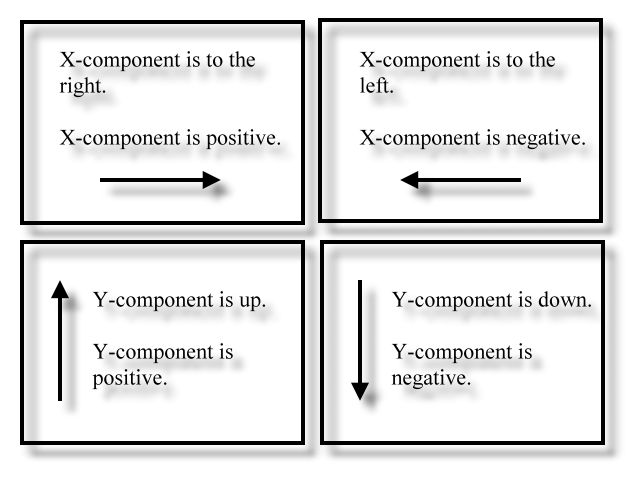﻿ Force Component Machine | Zona Land Education

# Force Component MachineFx = 0

Fy = 0

Click this button to see the computer code mathematics.

Here's the code that runs the above program.

You can change the code, if you like, and then click the following 'Reevaluate code' button. The program will then work as per your changes. Of course, your changes, especially random changes, can introduce errors, miscalculations, and browser crashes. If you need to get things back to their original condition, just reload this page using your browser's reload button.

The intention here is to conveniently show the inner workings of this program so that you understand how the diagram is drawn.

Click the 'Code' button again to close this section.

The Force Component Machine lets you visualize the x- and y-components of a two dimensional force vector. You can vary the size (magnitude) of the force and also point it in any direction on the (x, y) plane.

Click or tap the lesser and greater buttons to adjust the size and direction of the two dimensional force vector.

### The y-component is is in an lighter red.

The values for the x- and y-components can be positive or negative depending which way that the component is pointing. The following pictures describe this:The angle describing the direction of the two dimensional force vector is in degrees and is to be considered in standard position. In the above demonstration the angle is shown as a red filled arc symbol.

All numbers are rounded to two decimal points. Numbers such as '3.00' will be shown as '3'.

Related topics include:

Custom Search